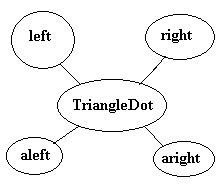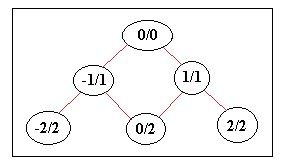PHP User Warning: fetch_template() calls should be replaced by the vB_Template class. Template name: bbcode_highlight in ..../includes/functions.php on line 4197
VS 2010 [RESOLVED] Pascal's triangle-VBForums

1. ##[RESOLVED] Pascal's triangle

hi,
I've done many attempts but still didn't succeed in making a logic for pascal's triangle, I want you guys to get me start this task and I'll code it myselflooking at Paul's thread, I don't understand how did the Paul do it.
http://www.vbforums.com/showpost.php...23&postcount=4
So, i need a logic, how would i start it?

thanksReply With Quote

2. ## Re: Pascal's triangle

Well if you look closely you can see the following:
1
12
123
1234
12345
So this is your base. On the other end you see that the inverted version of this is sticked to the one:
21
321
4321
54321
So it is somewhat like this:
1
Update number
Code:
```Public Function addSides(ByVal input As String, ByVal text As String) As String
Return StrReverse(text) & input & text
End Function```
I only have no idea why his code is so long. Is something special supposed to happen? xD

EDIT

Note: you need some sort of special formatting before every line to center the 1 correctly.Reply With Quote

3. ## Re: Pascal's triangleOriginally Posted by bergerkillerI only have no idea why his code is so long. Is something special supposed to happen? xD
Yep. that confused meok, i got take a look at your explanation but in the figure:sides of triangle would be 1, what about for numbers inside? (that's confusing for me)

i can understand that numbers adds up in a form of triangle and i can get a new number like: 1+1=2 then 1+2=3, 1+3=4 e.t.c
first i should make two right triangles and then concatenate them?

thanks..Reply With Quote

4. ## Re: Pascal's triangle

NeighbouringPositions (array index):Do as Paul did: start off with a 2-dimensional array of INTEGERs.
Code:
```Dim Count As Integer = 5
Dim grid(Count - 1)(Count - 1) As Integer
Fill(grid, Count / 2, 0)```
And make a recursive function fill this up.
Code:
```Public Sub Fill(ByRef input()() As Integer, ByVal x As Integer, ByVal y As Integer)
'check if input(x - 1, y - 1) is possible!!
input(x, y) += input(x - 1, y - 1)
'check if input(x + 1, y - 1) is possible!!
input(x, y) += input(x + 1, y - 1)
'neither? set 1
if input(x, y) = 0 Then input(x, y) = 1

'possible?
Fill(input, x - 1, y + 1)
'possible?
Fill(input, x + 1, y + 1)
End Sub```Reply With Quote

5. ## Re: Pascal's triangle

Try this:
vb.net Code:
`Private Function CreatePascalTriangle(ByVal numberOfRows As Integer) As List(Of Integer())        Dim triangle As New List(Of Integer())        For i As Integer = 0 To numberOfRows - 1            triangle.Add(New Integer(i) {})            triangle(i)(0) = 1            triangle(i)(i) = 1            If i > 1 Then                For j As Integer = 1 To i - 1                    triangle(i)(j) = triangle(i - 1)(j - 1) + triangle(i - 1)(j)                Next            End If        Next        Return triangle    End Function     Private Sub DisplayPascalTriangle(ByVal triangle As List(Of Integer()))        Dim midpoint As Integer = triangle.Count        Dim padding As Integer = 0        Dim line As String = String.Empty        Dim arr() As Integer = Nothing        Dim sb As New System.Text.StringBuilder        For row As Integer = 0 To triangle.Count - 1            padding = midpoint - row            arr = triangle(row)            For i As Integer = 0 To arr.GetUpperBound(0)                If i = 0 Then                    line = arr(i).ToString.PadLeft(padding) & " "                Else                    line &= arr(i).ToString & " "                End If            Next            sb.AppendLine(line)        Next        Debug.Write(sb.ToString)    End Sub     Private Sub Button1_Click(ByVal sender As System.Object, ByVal e As System.EventArgs) Handles Button1.Click        Dim triangle As List(Of Integer()) = CreatePascalTriangle(7)        DisplayPascalTriangle(triangle)    End Sub`Reply With Quote

6. ## Re: Pascal's triangle

will try itReply With Quote

####Posting Permissions

• You may not post new threads
• You may not post replies
• You may not post attachments
• You may not edit your posts
•

Featured